Lines and Rotational symmetry (Both)

Chapter 14 Class 7 Symmetry
Concept wise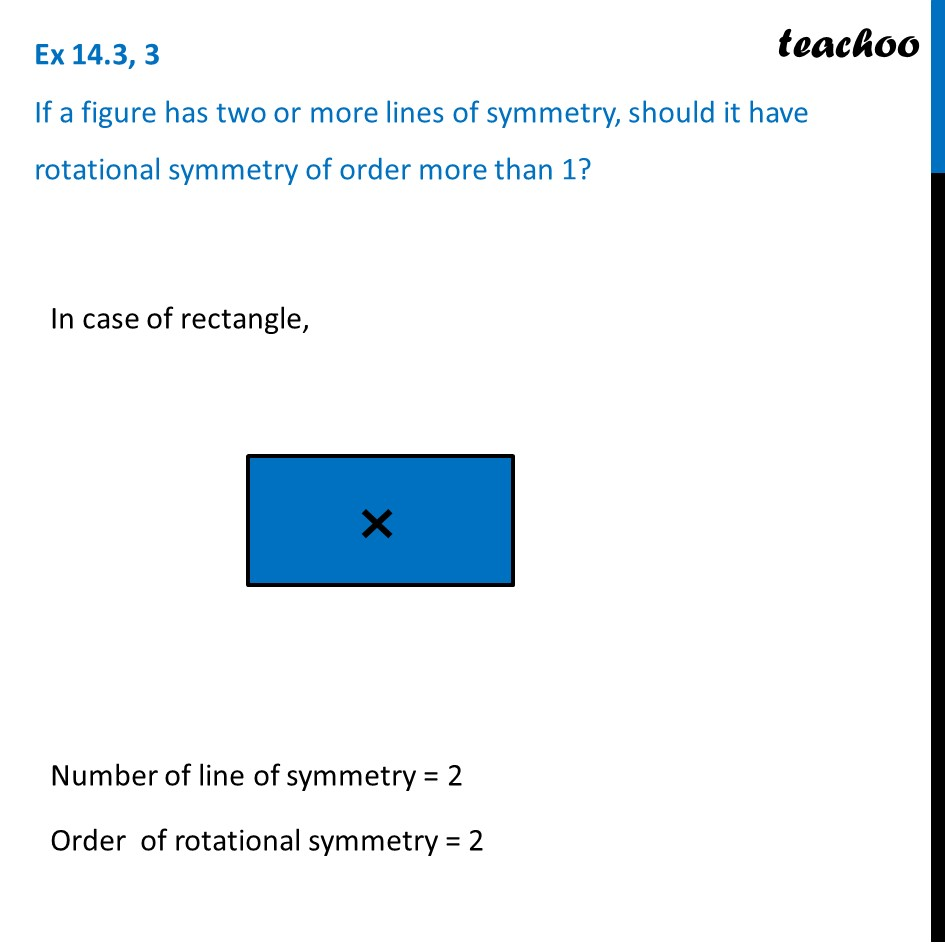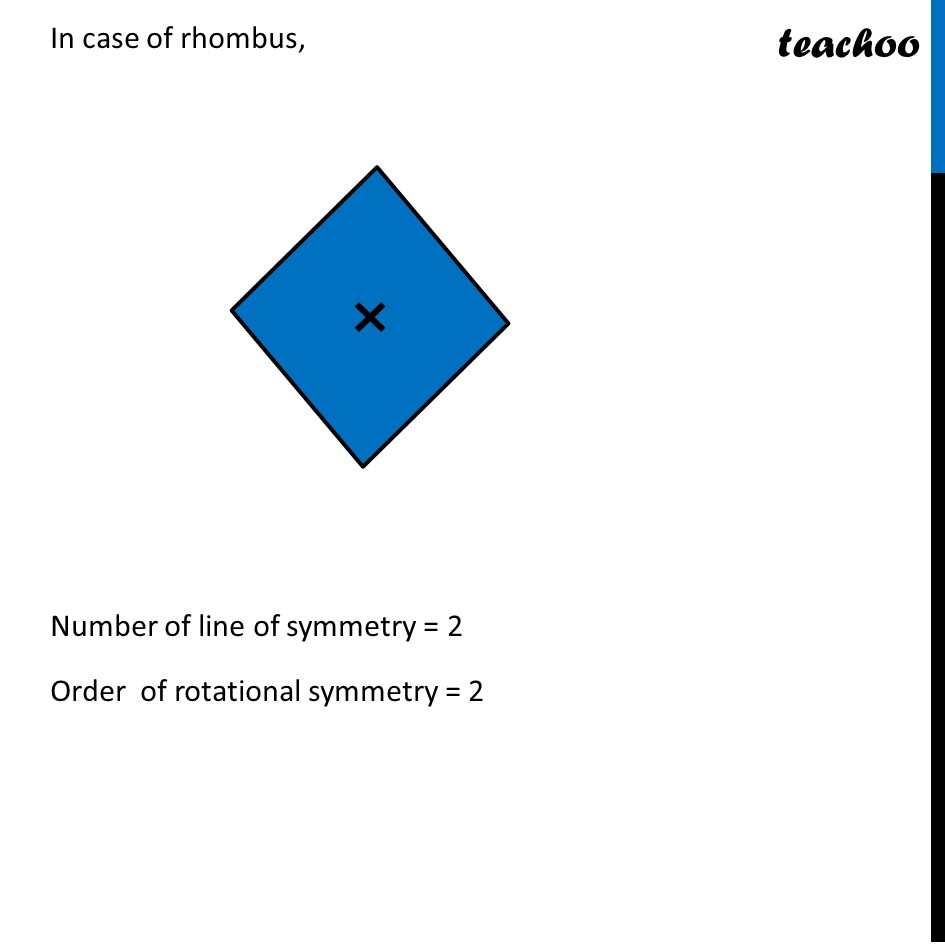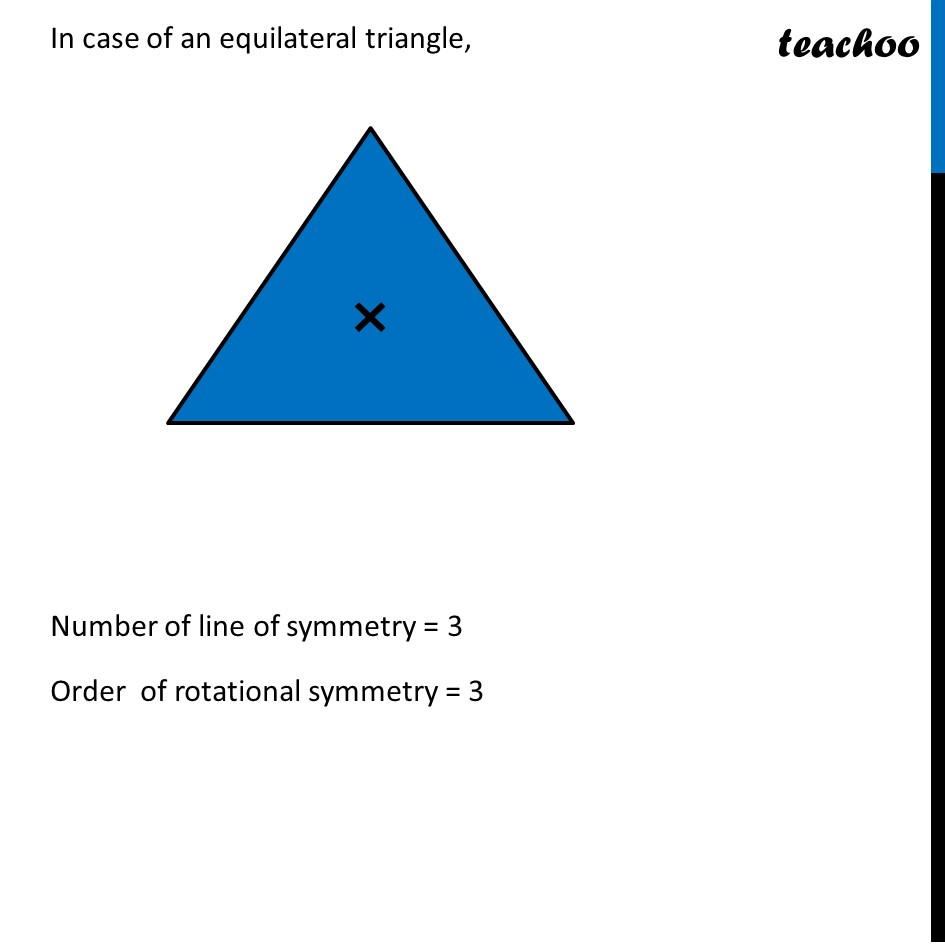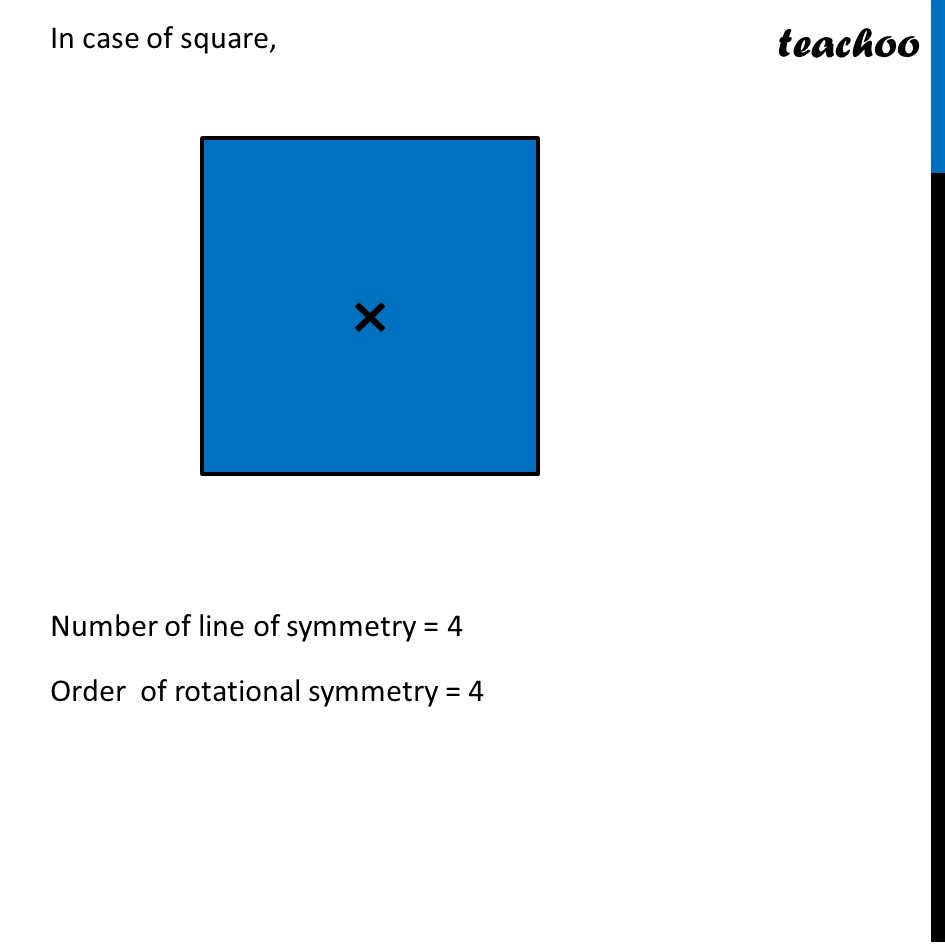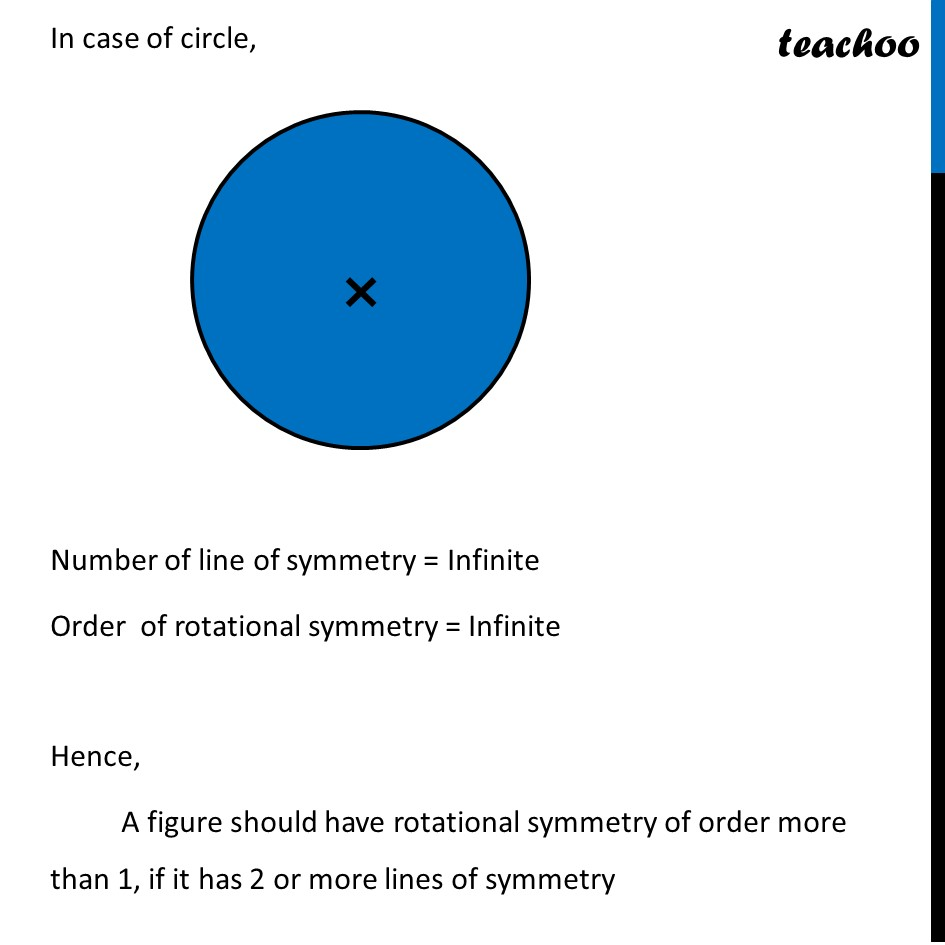Introducing your new favourite teacher - Teachoo Black, at only ₹83 per month

### Transcript

Ex 14.3, 3 If a figure has two or more lines of symmetry, should it have rotational symmetry of order more than 1?In case of rectangle, Number of line of symmetry = 2 Order of rotational symmetry = 2 In case of rhombus, Number of line of symmetry = 2 Order of rotational symmetry = 2 In case of an equilateral triangle, Number of line of symmetry = 3 Order of rotational symmetry = 3 In case of square, Number of line of symmetry = 4 Order of rotational symmetry = 4 In case of circle, Number of line of symmetry = Infinite Order of rotational symmetry = Infinite Hence, A figure should have rotational symmetry of order more than 1, if it has 2 or more lines of symmetry